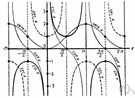# trigonometric function

Also found in: Thesaurus, Encyclopedia, Wikipedia.
Related to trigonometric function: exponential function, Inverse trigonometric function
trigonometric function
In a right triangle, the three main trigonometric functions are
sine θ = opposite / hypotenuse
cosine θ = adjacent / hypotenuse
tangent θ = opposite / adjacent.

## trigonometric function

n.
A function of an angle expressed as the ratio of two of the sides of a right triangle that contains that angle; the sine, cosine, tangent, cotangent, secant, or cosecant. Also called circular function.

## trigonometric function

n
1. (Mathematics) Also called: circular function any of a group of functions of an angle expressed as a ratio of two of the sides of a right-angled triangle containing the angle. The group includes sine, cosine, tangent, secant, cosecant, and cotangent
2. (Mathematics) any function containing only sines, cosines, etc, and constants

## trig′onomet′ric func′tion

n.
a function of an angle, as the sine or cosine, expressed as the ratio of the sides of a right triangle.
Also called circular function.
[1905–10]
trigonometric function
In a right triangle, the trigonometric functions are: sine θ = opposite/hypothenuse cosine θ = adjacent/hypothenuse tangent θ = opposite/adjacent

## trig·o·no·met·ric function

(trĭg′ə-nə-mĕt′rĭk)
A function of an angle, as the sine, cosine, or tangent, whose value is expressed as a ratio of two of the sides of the right triangle that contains the angle.
ThesaurusAntonymsRelated WordsSynonymsLegend:
 Noun 1trigonometric function - function of an angle expressed as a ratio of the length of the sides of right-angled triangle containing the anglecircular functionfunction, mapping, mathematical function, single-valued function, map - (mathematics) a mathematical relation such that each element of a given set (the domain of the function) is associated with an element of another set (the range of the function)sine, sin - ratio of the length of the side opposite the given angle to the length of the hypotenuse of a right-angled trianglearc sine, arcsin, arcsine, inverse sine - the inverse function of the sine; the angle that has a sine equal to a given numbercos, cosine - ratio of the adjacent side to the hypotenuse of a right-angled trianglearc cosine, arccos, arccosine, inverse cosine - the inverse function of the cosine; the angle that has a cosine equal to a given numbertangent, tan - ratio of the opposite to the adjacent side of a right-angled trianglearc tangent, arctan, arctangent, inverse tangent - the inverse function of the tangent; the angle that has a tangent equal to a given numbercotan, cotangent - ratio of the adjacent to the opposite side of a right-angled trianglearc cotangent, arccotangent, inverse cotangent - the inverse function of the cotangent; the angle that has a cotangent equal to a given numbersec, secant - ratio of the hypotenuse to the adjacent side of a right-angled trianglearc secant, arcsec, arcsecant, inverse secant - the inverse function of the secant; the angle that has a secant equal to a given numbercosec, cosecant - ratio of the hypotenuse to the opposite side of a right-angled trianglearc cosecant, arccosecant, inverse cosecant - the angle that has a cosecant equal to a given number
References in periodicals archive ?
Based on the derivation above, the angle calculation problem is transformed to root finding problem for polynomial, as there will be no trigonometric function of Snell's law.
As a result, two orthogonal intensity signals of [I.sub.x] [infinity] [I.sub.m,AC] * [I.sub.r] and [I.sub.y] [infinity] [I.sub.m,AC] * [I.sub.r][e.sup.j[pi]/2] can be represented as a trigonometric function.
First we assume that the superpotential [W.sub.1](x) is a trigonometric function which is
When [mu][lambda] > 0, the trigonometric function solution corresponding to the parameter values in Case 1 can be written as
which results in the following formula in terms of generalized trigonometric function defined in 
When I was studying Chemical Engineering at the University of Pennsylvania, I struggled with all the homework and lab work; not with the technical challenge but with the amount of time required to actually do the labor-intensive table look-ups for each trigonometric function used.
This equation requires division, support of floating point numbers and inverse trigonometric function which are not supported by VHDL .
They work on a trigonometric function based on the fact that your bow, the target, and the ground form a triangle whose unknown variable (the distance to the target) can be deduced.
When [e.sub.2] = 1, [e.sub.1] < 0, [e.sub.0] = 0, the following trigonometric function solution can be obtained:
On the basis of the Ritz method, [[xi].sub.j](x) can be approximately expressed as a known trigonometric function sin [[alpha].sub.j]x for the antisymmetric mode or cos [[beta].sub.j]x for the symmetric mode, in which [[alpha].sub.j], [[beta].sub.j] are the known constants.
In this paper, monotonicity of data is retained by developing constraints on free parameters in the specification of rational trigonometric function and bicubic blended function.
We claim that the tanh method can be extended by replacing tanh function with some generalized functions f(x), such as polynomial function, trigonometric function and Jacobi elliptical function.

Site: Follow: Share:
Open / Close